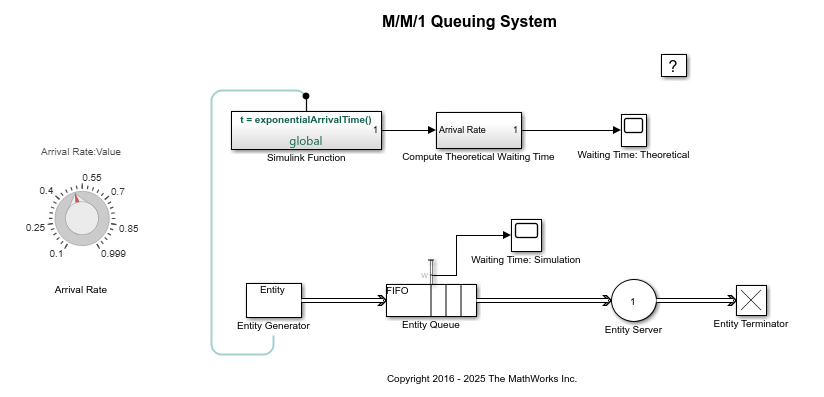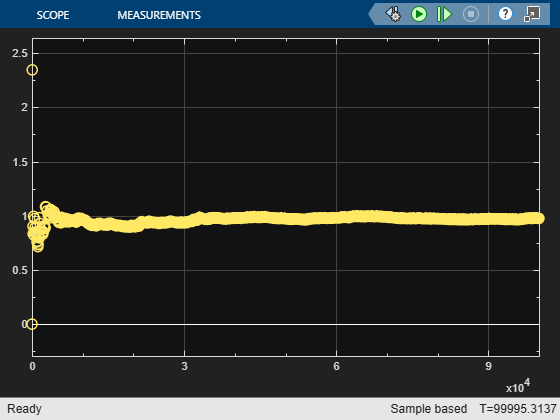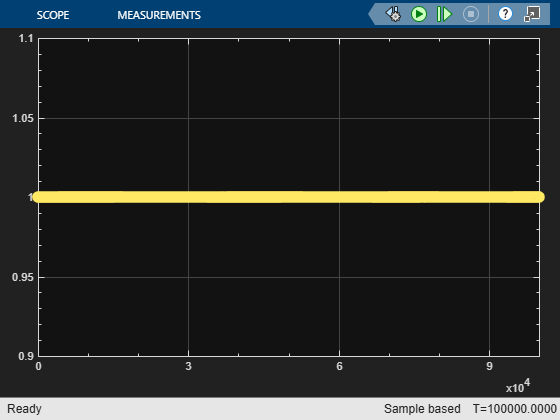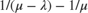# M/M/1 Queuing System

### Overview

This example shows how to model a single-queue single-server system with a single traffic source and an infinite storage capacity. In the notation, the M stands for Markovian; M/M/1 means that the system has a Poisson arrival process, an exponential service time distribution, and one server. Queuing theory provides exact theoretical results for some performance measures of an M/M/1 queuing system and this model makes it easy to compare empirical results with the corresponding theoretical results.

### Structure

The model includes the components listed below:

• Entity Generator block: Models a Poisson arrival process by generating entities (also known as "customers" in queuing theory).

• Simulink Function exponentialArrivalTime(): Returns data representing the interarrival times for the generated entities. The interarrival time of a Poisson arrival process is an exponential random variable.

• Entity Queue block: Stores entities that have yet to be served in FIFO order

• Entity Server block: Models a server whose service time has an exponential distribution.### Results and Displays

The model includes these visual ways to understand its performance:

• Scopes labeled "Waiting Time: Theoretical" and "Waiting Time: Simulation" showing the theoretical and empirical values of the waiting time in the queue, on a single set of axes. You can use this plot to see how the empirical values evolve during the simulation and compare them with the theoretical value.

• A scope labeled "Server Utilization" showing the utilization of the single server over the course of the simulation.### Theoretical Results

Queuing theory provides the following theoretical results for an M/M/1 queue with an arrival rate ofand a service rate of:

• Mean waiting time in the queue =The first term is the mean total waiting time in the combined queue-server system and the second term is the mean service time.

• Utilization of the server =### Experimenting with the Model

Move the Arrival Rate knob during the simulation and observe the change in the simulation results

### References

 Kleinrock, Leonard, Queueing Systems, Volume I: Theory, New York, Wiley, 1975.